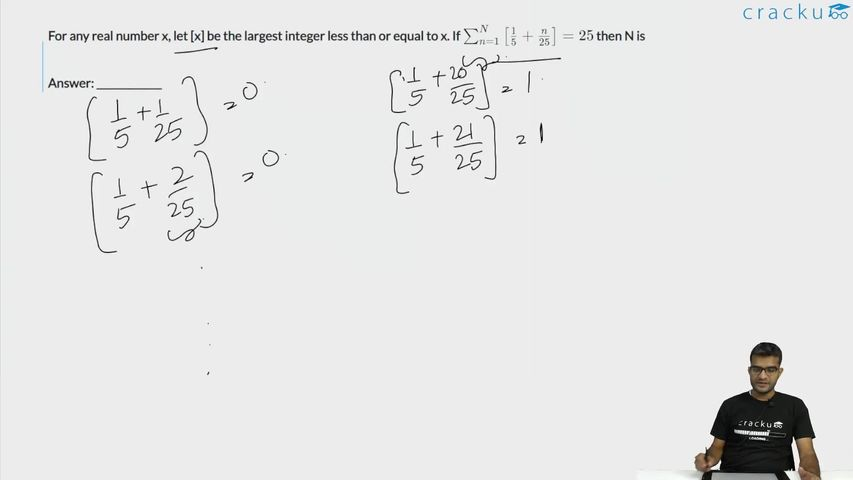Question 66

# For any real number x, let [x] be the largest integer less than or equal to x. If $$\sum_{n=1}^N \left[\frac{1}{5} + \frac{n}{25}\right] = 25$$ then N is

Solution

It is given,

$$\Sigma_{n=1}^N\ \left[\frac{1}{5}+\frac{n}{25}\right]=25$$

$$\Sigma_{n=1}^N\ \left[\frac{5+n}{25}\right]=25$$

For n = 1 to n = 19, value of function is zero.

For n = 20 to n = 44, value of function will be 1.

44 = 20 + n - 1

n = 25 which is equal to given value.

This implies N = 44

### View Video Solution• All Quant CAT Formulas and shortcuts PDF
• 30+ CAT previous papers with solutions PDF

##### om bikash

4 months, 3 weeks ago

Nice

##### om bikash

4 months, 3 weeks ago

Hare Krishna hare Krishna Krishna Krishna hare hare
Hare ram hare ram ram ram hare hare